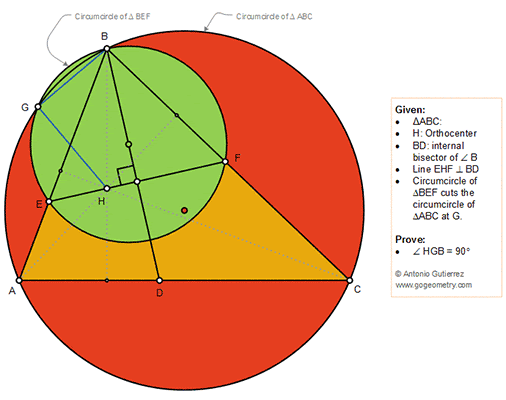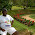Wednesday, September 5, 2018

Geometry Problem 1384: Triangle, Orthocenter, Circle, Circumcircle, Angle Bisector, 90 Degree

Geometry Problem. Post your solution in the comment box below.
Level: Mathematics Education, High School, Honors Geometry, College.

Details: Click on the figure below.1.Triangle AEH is similar to Triangle CFH by AAA, we get EH/FH=AE/CF ....1
Similarly Tr. AGE is similar to Tr. CGF by AAA, We have EG/FG=AE/CF ....2
Using eq 1 & 2 we get EG/FG=EH/FH, hence GH must be bisector of Angle EGF, we have Angle EGH=B/2, Since Angle EGB=90+(B/2), Angle HGB=90.

2.1.